Wir verwenden Google für unsere Suche. Mit Klick auf „Suche aktivieren“ aktivieren Sie das Suchfeld und akzeptieren die Nutzungsbedingungen.

# New in SG-Lib 4.2

###### symbforwkine(a,b,WL)- returns a forward kinematic calculation from a symbolics links list description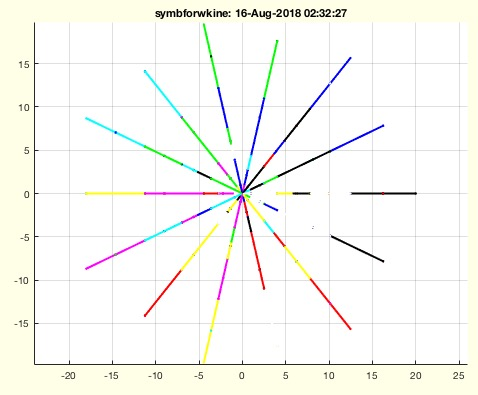% symbforwkine(a,b,WL) - returns a forward kinematic calculation from a symbolics links list description% (by Tim Lueth, VLFL-Lib, 2018-AUG-16 as class: EXPERIMENTS)% % This fnctn takes a cell list "b" of symbolic 4x4 matrices with exactly % one degree of freedom (1DoF). They are generated, for example, by the % fnctn SGTofDHset.% These are now converted by matrix multiplication into fnctns of the % endpoints of a kinematic chain. The first 4x4 matrix has 1Dof, the % second matrix then 2DoF etc. This cell list Ti of the symbolic % equations for calculating the endpoints of the kinematic chain is then % converted into a cell list H of handeles of the corresponding matlab % fnctns. The fnctns in H can then be used to calculate the endpoints % numerically. Optionally, an angle list is generated, which is a % permutation of angle positions at each DoF. (Status of: 2018-08-16)% % Introduced first in SolidGeometry 4.2% % See also: SGTofDHset% % [Ti,LL,H]=symbforwkine(a,b,[WL])% === INPUT PARAMETERS ===% a: unused yet% b: cell list of n symbolics symbolic HT matrices x of 1doF rotational % links % WL: List of k angles of n links [k x l]% === OUTPUT RESULTS ======% Ti: cell list of n symbolic HT matrices of b link end points% LL: matrix [n x 3 x k]% H: matlab fnctn handles the calculation of Ti% % EXAMPLE: % SGTofDHset([20 0 pi/2 0 ;0 10 pi 0;0 10 0 pi/2;10 0 pi/4 0; 10 0 pi/4 % 0]); show% [a,b]=SGTofDHset([20 0 pi/2 0 ;0 10 pi 0;0 10 0 pi/2;10 0 pi/4 0; 10 0 % pi/4 0]);% symbforwkine(a,b);% [e,f,g]=symbforwkine(a,b,2)% e{:},g{:}% % See also: SGTofDHset% % % Copyright 2018 Tim C. Lueth
###### exp_2018_08_14_forwkinsym(a,b,WL)- EXPERIMENT that returns a forward kinematic calculation from a symbolics links list description% exp_2018_08_14_forwkinsym(a,b,WL) - EXPERIMENT that returns a forward kinematic calculation from a symbolics links list description% (by Tim Lueth, VLFL-Lib, 2018-AUG-14 as class: EXPERIMENTS)% % will be renamed into symbforwkine (Status of: 2018-08-16)% % Introduced first in SolidGeometry 4.2% % [Ti,LL,H]=exp_2018_08_14_forwkinsym(a,b,[WL])% === INPUT PARAMETERS ===% a: unused yet% b: cell list of n symbolics symbolic HT matrices x of 1doF rotational % links % WL: List of k angles of n links [k x l]% === OUTPUT RESULTS ======% Ti: cell list of n symbolic HT matrices of b link end points% LL: matrix [n x 3 x k]% H: matlab fnctn handles the calculation of Ti% % EXAMPLE: % SGTofDHset([20 0 pi/2 0 ;0 10 pi 0;0 10 0 pi/2;10 0 pi/4 0; 10 0 pi/4 % 0]);% [a,b]=SGTofDHset([20 0 pi/2 0 ;0 10 pi 0;0 10 0 pi/2;10 0 pi/4 0; 10 0 % pi/4 0]);% exp_2018_08_14_forwkinsym(a,b);% [e,f,g]=exp_2018_08_14_forwkinsym(a,b,2)% e{:},g{:}% % % Copyright 2018 Tim C. Lueth
###### SGofVLalphaShape(VL,al)- returns the alphashape of a vertex list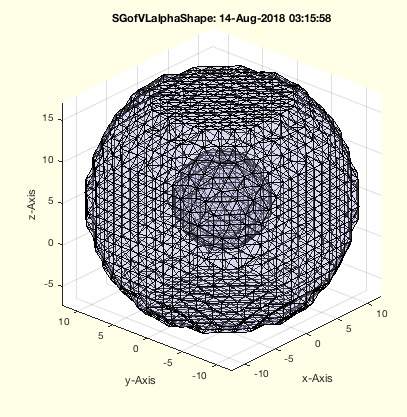% SGofVLalphaShape(VL,al) - returns the alphashape of a vertex list% (by Tim Lueth, VLFL-Lib, 2018-AUG-14 as class: TETRAHEDRONS)% % Introduced first in SolidGeometry 4.2% % See also: alphaShape, alphaTriangulation, triangulation, freeBoundary% % SG=SGofVLalphaShape(VL,[al])% === INPUT PARAMETERS ===% VL: Vertex List% al: optional alpha radius% === OUTPUT RESULTS ======% SG: Solid Geometry of the Alpha Shape% % EXAMPLE: % [a,b,c,d,e,f]=invkinrplan3(5,10,5); VL=f(e,:); SGfigure; % VLplot(VL,'.'); view(-30,30)% SGofVLalphaShape(VL)% % % See also: alphaShape, alphaTriangulation, triangulation, freeBoundary% % % Copyright 2018 Tim C. Lueth
###### isrealr(VL)- returns whether all elements on a row are real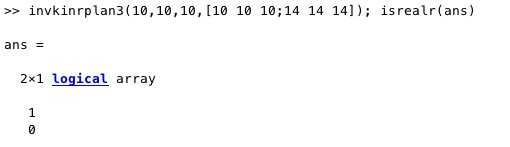% isrealr(VL) - returns whether all elements on a row are real% (by Tim Lueth, VLFL-Lib, 2018-AUG-14 as class: ANALYTICAL GEOMETRY)% % similar concept to norm and normc and normr (Status of: 2018-08-14)% % Introduced first in SolidGeometry 4.2% % See also: isreal% % ri=isrealr(VL)% === INPUT PARAMETERS ===% VL: vector list with rows and columns [n x m]% === OUTPUT RESULTS ======% ri: index list; r(i)==true if all elements of VL(i,:) = real% % EXAMPLE: % invkinrplan3(10,10,10,[10 10 10;14 14 10]); isrealr(ans)% invkinrplan3(10,10,10,[10 10 10;14 14 14]); isrealr(ans)% % See also: isreal% % % Copyright 2018 Tim C. Lueth
###### exp_2018_08_12_torsion (VL,wr,ccw,CPL,n)- EXPERIMENT to create torsion ropes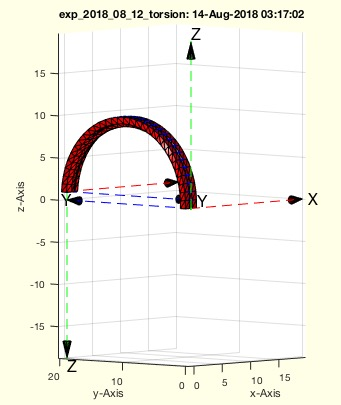% exp_2018_08_12_torsion (VL,wr,ccw,CPL,n) - EXPERIMENT to create torsion ropes% (by Tim Lueth, VLFL-Lib, 2018-AUG-13 as class: EXPERIMENTS)% % Several fnctns have already been designed by the author to extrude a % body along a path: SGofCPLCVVLR, SGcontourtube, SGcontourtube2, % SGofCPLtransT. If the cross-sectional contour CPL is always to be % perpendicular to the path and a rotation about the z-axis is to be % realized at the same time (torsion), the body is constricted. This can % only be avoided by deviating from the original path and placing a helix % around the path. This extends the distance and reduces constriction. % The rotation around ez is connected with a curvature around ex/ey. This % happens, for example, when shooting up a sheet while sailing or a % climbing rope. The inverse kinematics of a 6D robot also allows this % problem to be solved. % This experiment illustrates different couplings of a curvature with the % rotation around the z-axis. (Status of: 2018-08-16)% % Introduced first in SolidGeometry 4.2% % exp_2018_08_12_torsion([VL,wr,ccw,CPL,n])% === INPUT PARAMETERS ===% VL: 2 Points as list {x1 y1 z1; x2 y2 z2]% wr: rotation angle% ccw: [fx fy fz] value for cw or ccw for rotation x rotation y and % rotation z; default is [1 1 1]% CPL: cross section contour ; default is CPLSample(33)% n: number of rotations per pi% % EXAMPLE: % testfunctTL('exp_2018_08_12_torsion('''',#3,[#1 #2]);',[-1 0 +1],[-1 0 % +1],1*[pi -pi])% % % Copyright 2018 Tim C. Lueth
###### SGofCPLtransT(CPL,T1,T2)- returns a solid from a 2D contour and a list of frames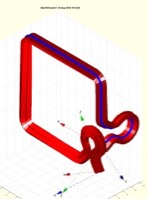% SGofCPLtransT(CPL,T1,T2) - returns a solid from a 2D contour and a list of frames % (by Tim Lueth, VLFL-Lib, 2018-AUG-13 as class: SURFACES)% % In combination with TLofCVL, this fnctn will replace in future mostly % all other fnctns such as: SGofCPLCVLR, SGcontourtube, SGcontourtube2, % SGof2T, SGof2SGT. (Status of: 2018-08-16)% % Introduced first in SolidGeometry 4.2% % See also: TLofCVL, SGofCPLCVLR, SGcontourtube, SGcontourtube2, SGof2T, % SGof2SGT% % % [SG,FLS,FLE,FLW,TS,TE]=SGofCPLtransT(CPL,T1,[T2])% === INPUT PARAMETERS ===% CPL: Closed Polygon List separated by nan% T1: Frame 1 [4 x 4] or List of frames [4 x 4 x n]% T2: Frame 2 [4 x 4], optional% === OUTPUT RESULTS ======% SG: Solid Geometry SG.VL, SG.FL% FLS: Facets of the start cover% FLE: Facets of the end cover% FLW: Facets of the contour wall% TS: starting frame% TE: ending frame% % EXAMPLE: % SGofCPLtransT(CPLsample(13),eye(4),TofR(rot(0,0,pi/20),[0 0 40]));% TL=TLofCVL(VLsample(4),2,eye(3),rot(0,0,pi/2),'rad'); % SG=SGofCPLtransT(CPLsample(27)/20,TL); SGfigure(SG); view(-30,30);% % See also: TLofCVL, SGofCPLCVLR, SGcontourtube, SGcontourtube2, SGof2T, % SGof2SGT% % % % Copyright 2018 Tim C. Lueth
###### TLofCVL(CVL,R,T1,T2,ty,tor)- returns a list of frames that connect a start frame and an end frame along a spatial contour% TLofCVL(CVL,R,T1,T2,ty,tor) - returns a list of frames that connect a start frame and an end frame along a spatial contour% (by Tim Lueth, VLFL-Lib, 2018-AUG-11 as class: ANALYTICAL GEOMETRY)% % This fnctn creates a list of HT frames for a vertex list, a start frame % and an end frame as well as a radius specification with which a % transition between start and end frames can be achieved. First, % VLradialEdges2T adds radii to the contour in order to avoid strong % curvature changes. The rotation by ez remains between the Start Frame % and the End Frame. For this purpose support points are inserted on long % straight lines. Then the necessary rotation error between the start % frame and the end frame is determined in relation to the broken curve % surface normal (VLedgeNormal). The required rotatation is equally % distributed between start and end points (Status of: 2018-08-16)% % Introduced first in SolidGeometry 4.2% % See also: SGofCPLtransT, VLradialEdges2T, VLedgeNormal% % Ti=TLofCVL([CVL,R,T1,T2,ty,tor])% === INPUT PARAMETERS ===% CVL: Closed Contour line% R: Radius% T1: Start Frame% T2: End Frame% ty: type 'rad', 'rmax', 'tan'; default is 'rad'; see VLradialEdges2T% tor: true=>torsion; false=>no torsion; default is true% === OUTPUT RESULTS ======% Ti: List of HT-Matrixes (4 x 4 x n] for n vertices% % EXAMPLE: % TLofCVL(VLsample(7),2,eye(3),rot(0,0,pi/2),'rad');TL=ans;% TLofCVL(VLsample(7),2,eye(3),rot(0,0,pi/2),'rmax');TL=ans;% TLofCVL(VLsample(7),2,eye(3),rot(0,0,pi/2),'tan');TL=ans;% TLofCVL(VLsample(3),2,eye(3),rot(0,0,pi/2),'tan',true);TL=ans;% TLofCVL(VLsample(3),2,eye(3),rot(0,0,pi/2),'tan',false);TL=ans;% % See also: SGofCPLtransT, VLradialEdges2T, VLedgeNormal% % % Copyright 2018 Tim C. Lueth
###### PLtangentcirc3(PL,R,maxR)- returns the a circ segment between p1 and p3 with radius R% PLtangentcirc3(PL,R,maxR) - returns the a circ segment between p1 and p3 with radius R% (by Tim Lueth, VLFL-Lib, 2018-AUG-10 as class: ANALYTICAL GEOMETRY)% % A warning of thrown if the fnctn is called without R as 3rd output % argument in contrast to PLtangentcirc, this fnctn calculates an outer % radius solution instead of throwing a warning (Status of: 2018-08-10)% % Introduced first in SolidGeometry 4.2% % See also: VLradialEdges, VLtangentcirc, PLtangentcirc, PLradialEdges, % VLradialEdges2T% % [PLE,p2x,R]=PLtangentcirc3([PL,R,maxR])% === INPUT PARAMETERS ===% PL: three points [p1; p2; p3]% R: Desired Radius% maxR: option to maximize the radius% === OUTPUT RESULTS ======% PLE: Point List to replace 2nd point of the list% p2x: Center Points of the Radius% R: Radius used in PLtangentcirc% % EXAMPLE: try different examples% PLtangentcirc (CPLsample(1),5);% PLtangentcirc (PLsample(5)); % warning% [~,~,r]=PLtangentcirc (PLsample(5)) % No warning% % See also: VLradialEdges, VLtangentcirc, PLtangentcirc, PLradialEdges, % VLradialEdges2T% % % Copyright 2018 Tim C. Lueth
###### sphere_line_intersection(l1,l2,sp,r)- returns the crossing points of a sphere and a straight line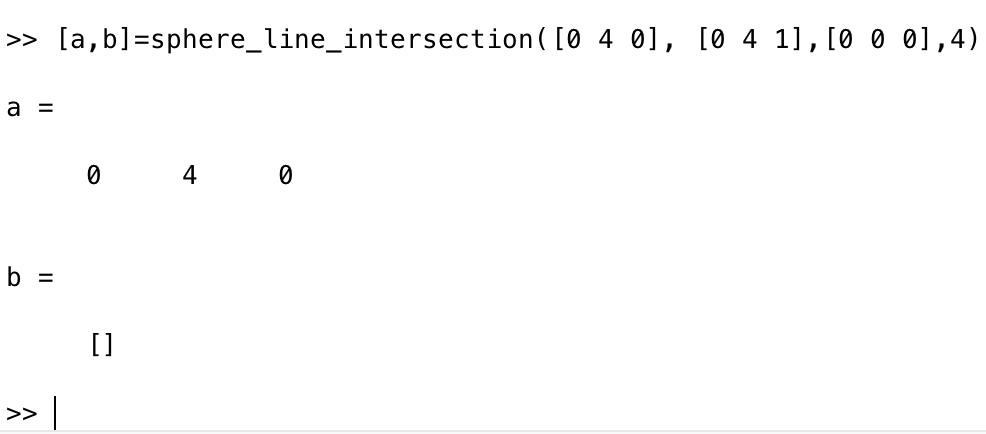% sphere_line_intersection(l1,l2,sp,r) - returns the crossing points of a sphere and a straight line% (by Paul Bourke & Campbell Barton, VLFL-Lib, 2018-AUG-08 as class: % ANALYTICAL GEOMETRY)% % This implementation is taken from the python 3.2 code:% http://paulbourke.net/geometry/circlesphere/% http://paulbourke.net/geometry/circlesphere/sphere_line_intersection.py% and transfered to Matlab by Tim Lueth% at Greg Hager's House in Mt. Crested Butte (Status of: 2018-08-08)% % See also: crosscircline% % [p1,p2]=sphere_line_intersection(l1,l2,sp,r)% === INPUT PARAMETERS ===% l1: line point 1 [ x y z]% l2: line point 2 [ x y z]% sp: sphere center coordinate [ x y z]% r: radius R% === OUTPUT RESULTS ======% p1: return crossing point 1 if exist or []% p2: return crossing point 2 if exist or []% % EXAMPLE: % [a,b]=sphere_line_intersection([0 0 0], [0 0 1],[0 0 0],4) % two % crossing points% [a,b]=sphere_line_intersection([0 4 0], [0 4 1],[0 0 0],4) % one % crossing point% % See also: crosscircline%
###### crosscircline(C,R,p,ez)- returns the cross points of a circle and a straight line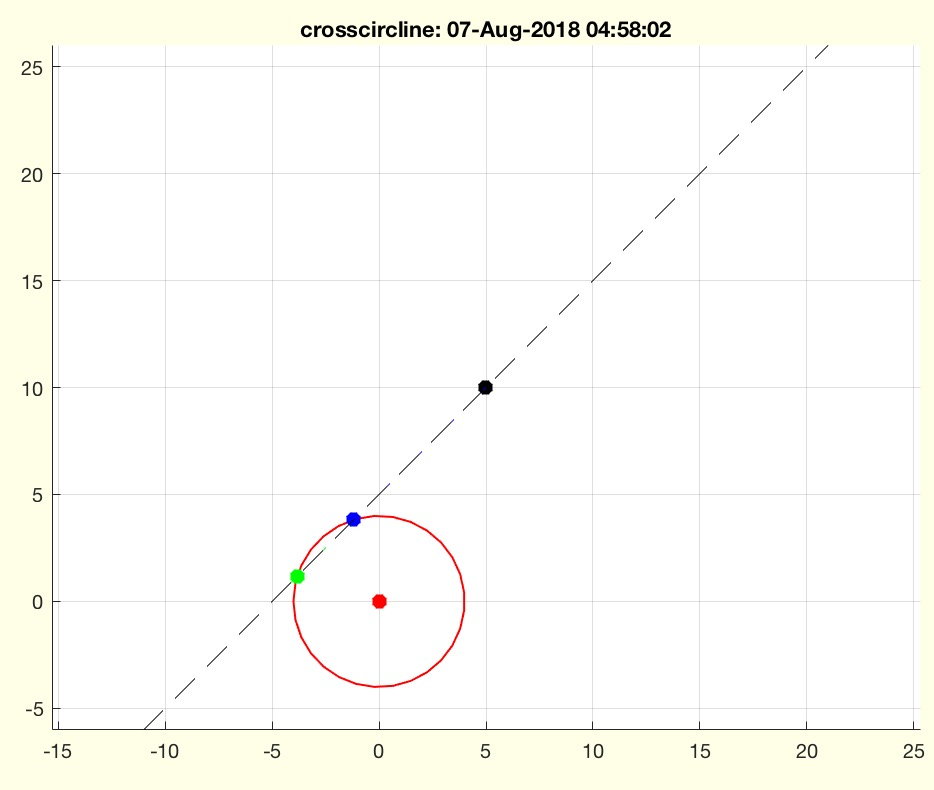% crosscircline(C,R,p,ez) - returns the cross points of a circle and a straight line% (by Tim Lueth, Video-Lib, 2018-AUG-06 as class: ANALYTICAL GEOMETRY)% % The solution was created using the Symbolics toolbox. Therefor, the % solutions may not correspond.% syms cx cy w R y0 k y1 x0 k x1% r=solve(cx + cos (w) * R ==y0 + k * y1, cy +sin (w) * R == x0 + k * % x1,k,w,'Real',true)% (Status of: 2018-08-08)% % Introduced first in SolidGeometry 4.2% % See also: tangent2circ, circcirc, cross2circ, PLof3dist, PLchordof2PR, % crossz, PLcross2Lines% % [P,K,W]=crosscircline(C,R,p,ez)% === INPUT PARAMETERS ===% C: Center of circle [x y]% R: Radius of circle% p: point on straight line% ez: direction of straight line% === OUTPUT RESULTS ======% P: List of 2 Points% K: List of 2 K values% W: List of two angle% % EXAMPLE: % testfunctTL('crosscircline([0 0],4,[#1 #2],[.5 1])',[-5 5],[-3 2]);% testfunctTL('crosscircline([0 0],6,[#1 #2],[.5 1])',[-5 5],[-3 2]);% crosscircline([0 0],4,[0 2],[1 1]) % two solutions% crosscircline([0 0],4,[5 5],[1 1]) % two solutions% crosscircline([0 0],4,[4 10],[0 1]) % one Solution% crosscircline([0 0],4,[5 10],[0 1]) % No Solution% % See also: tangent2circ, circcirc, cross2circ, PLof3dist, PLchordof2PR, % crossz, PLcross2Lines% % % Copyright 2018 Tim C. Lueth
###### exp_2018_08_04_jointsubstraction- EXPERIMENT TO CREATE A SUBSTRACTION BODY FOR CREATING JOINTS FROM BARS% exp_2018_08_04_jointsubstraction - EXPERIMENT TO CREATE A SUBSTRACTION BODY FOR CREATING JOINTS FROM BARS% (by Tim Lueth, VLFL-Lib, 2018-AUG-04 as class: EXPERIMENTS)% % Just before flying out to Prof. Greg Hager (JHU) in Colorado (Status % of: 2018-08-04)% % Introduced first in SolidGeometry 4.2% % F=exp_2018_08_04_jointsubstraction% === OUTPUT RESULTS ======% F: Final Substraction Body% % % Copyright 2018 Tim C. Lueth
###### SGsimpleNDItracker(d,H,A)- creates a tracker for NDI spheres using a distance list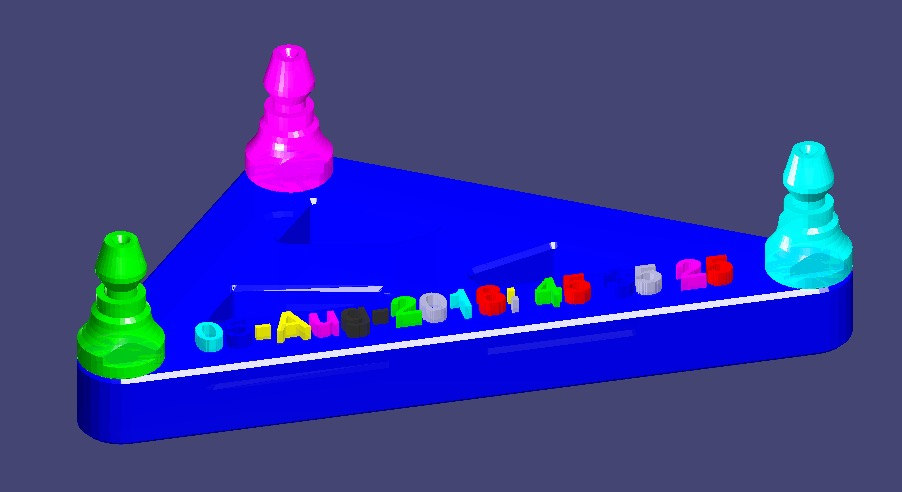% SGsimpleNDItracker(d,H,A) - creates a tracker for NDI spheres using a distance list% (by Tim Lueth, VLFL-Lib, 2018-AUG-01 as class: MODELING PROCEDURES)% % Introduced first in SolidGeometry 4.2% % See also: SGsimpleHinge% % [SG,cp]=SGsimpleNDItracker(d,[H,A])% === INPUT PARAMETERS ===% d: distance list such as [40 50 60]% H: height of the tracker% A: Geometry of the mounting posts% === OUTPUT RESULTS ======% SG: Resulting Solid Geoemtry% cp: center point% % EXAMPLE: % loadweb NDI_STLs.mat% SGsimpleNDItracker([80 50 40],'',NDI_mountingpost);% A=SGsimpleNDItracker([80 50 40],[],NDI_mountingpost);% B=SGbending(A,40); SGfigure(B)% % See also: SGsimpleHinge% % % Copyright 2018 Tim C. Lueth
###### PLchordof2PR(PL,R,)- returns a radial segment for a given chord and a radius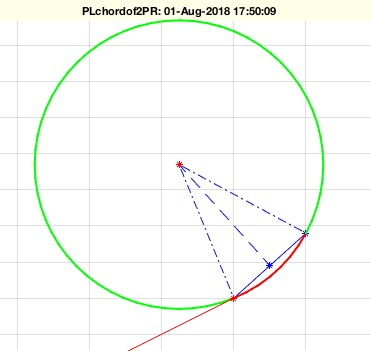% PLchordof2PR(PL,R,) - returns a radial segment for a given chord and a radius% (by Tim Lueth, VLFL-Lib, 2018-AUG-01 as class: ANALYTICAL GEOMETRY)% % Introduced first in SolidGeometry 4.2% % See also: cross2circ, PLof3dist, tangent2circ, crossz% % [PL1,PL2,m1,m2]=PLchordof2PR(PL,R,[])% === INPUT PARAMETERS ===% PL: [starting point of chord; end point of chord]% R: Radius% === OUTPUT RESULTS ======% PL1: short angle (red)% PL2: long angle (green)% m1: center on left side% m2: center on right side% % EXAMPLE: % PLchordof2PR([0 0;10 0],5)% PLchordof2PR([0 0;10 0],9)% PLchordof2PR([0 0; 10 9],20,[4,2]);% % See also: cross2circ, PLof3dist, tangent2circ, crossz% % % Copyright 2018 Tim C. Lueth
###### SGboxpacking(SG,tstr,dim,turn)- combines SGpacking and SGboxing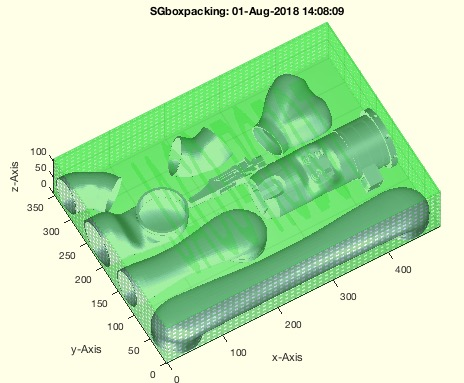% SGboxpacking(SG,tstr,dim,turn) - combines SGpacking and SGboxing% (by Tim Lueth, VLFL-Lib, 2018-AUG-01 as class: AUXILIARY PROCEDURES)% % Auxiliaray fnctn to prepare print jobs for Selective Laser Sintering % (Status of: 2018-08-01)% % Introduced first in SolidGeometry 4.2% % See also: SGpacking, SGboxing, SGpatternXYZ, SGcopyrotZ, SGarrangeSG, % SGarrangeSGC, SGCaddSGn, SGCaddSG% % [SGC,SGA,SGB]=SGboxpacking(SG,[tstr,dim,turn])% === INPUT PARAMETERS ===% SG: SG or SGcs to pack and box% tstr: text string for cover% dim: Box dimensions for printing% turn: false==keep orientation; true==optimize space; default is true% === OUTPUT RESULTS ======% SGC: complete print job SG% SGA: packaged solids of SG% SGB: box aroound SGA% % EXAMPLE: % loadweb JACO_robot.mat; SGboxpacking(JACO,'JACO-ROBOT',[500 500 500]);% SGboxpacking(JACO,'JACO-ROBOT',[500 500 500],false); % % % See also: SGpacking, SGboxing, SGpatternXYZ, SGcopyrotZ, SGarrangeSG, % SGarrangeSGC, SGCaddSGn, SGCaddSG% % % Copyright 2018 Tim C. Lueth
###### SGofVLFLSGc(SGc)- returns only the Solid Geometry of structs, Solid, Vertex or Facet list% SGofVLFLSGc(SGc) - returns only the Solid Geometry of structs, Solid, Vertex or Facet list% (by Tim Lueth, VLFL-Lib, 2018-AUG-01 as class: SURFACES)% % all frames etc are lost. This fnctn is used to structure several % printjobs% Could be extended to handels, pde models etc. (Status of: 2018-08-01)% % Introduced first in SolidGeometry 4.2% % See also: SGpacking% % SG=SGofVLFLSGc(SGc)% === INPUT PARAMETERS ===% SGc: Any kind of SG: A.SG, A{}, A.VL,A.FL% === OUTPUT RESULTS ======% SG: Struct only with fields SG.Vl and SG.FL% % EXAMPLE: % load JACO_robot.mat% SGofVLFLSGc(JACO)% % % See also: SGpacking% % % Copyright 2018 Tim C. Lueth
###### PLtransC(PL,C,w)- rotates a point list around a center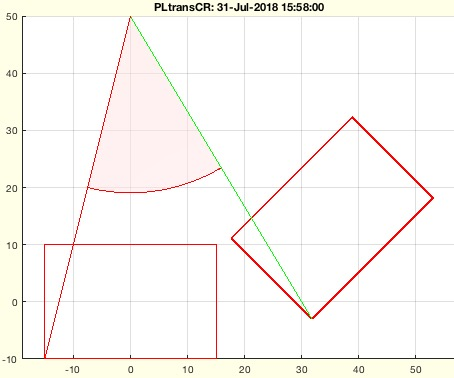% PLtransC(PL,C,w) - rotates a point list around a center% (by Tim Lueth, VLFL-Lib, 2018-JUL-31 as class: CLOSED POLYGON LISTS)% % Introduced first in SolidGeometry 4.2% % See also: PLtransP, PLtransR, PLtrans, PLtrans0, PLtrans1, PLtransT% % PL=PLtransC(PL,C,w)% === INPUT PARAMETERS ===% PL: Point List [nx2]% C: Center of rotation default is zero% w: angle of rotation default is pi/2% === OUTPUT RESULTS ======% PL: Resulting Point list% % EXAMPLE: % PLtransC(PLsquare(30,20),[0 50],pi/4)% % See also: PLtransP, PLtransR, PLtrans, PLtrans0, PLtrans1, PLtransT% % % Copyright 2018 Tim C. Lueth
###### SGsimpleHinge(H,D,X,sl,ch,oa,ba,Ri);- returns a simple swivel hinge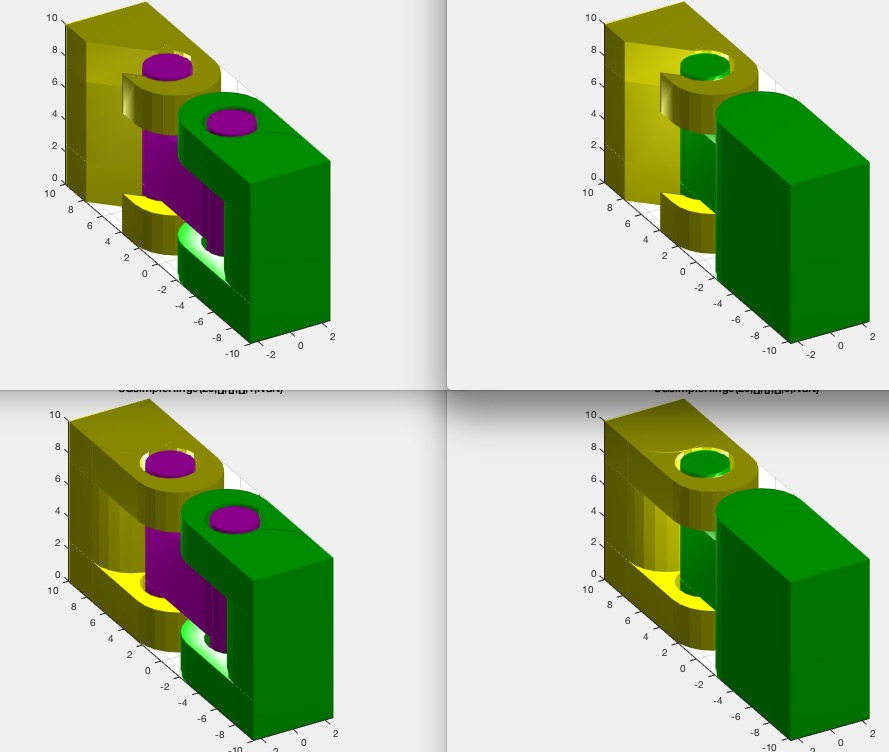% SGsimpleHinge(H,D,X,sl,ch,oa,ba,Ri); - returns a simple swivel hinge% (by Tim Lueth, VLFL-Lib, 2018-JUL-30 as class: MODELING PROCEDURES)% % First version as tutorial for Alexandra Mercader (Status of: 2018-08-01)% % Introduced first in SolidGeometry 4.2% % See also: SGmodelLink2% % [SG,SG1,SG2,SG3]=SGsimpleHinge([H,D,X,sl,ch,oa,ba,Ri]);% === INPUT PARAMETERS ===% H: height in z% D: depth in y; default is H/2% X: Depth in x; default is D/2% sl: slot size; default is 0.3mm for SLS% ch: chain; default is false% oa: opening angle starting at 5*pi/4; default is []==closed% ba: angle intervall [intervall, start]; not supported yet% Ri: inner Radius; default is R/1.5% === OUTPUT RESULTS ======% SG: Complete Solid Geometry% SG1: Upper Part, 'yellow% SG2: Lower Part% SG3: Middle Part% % EXAMPLE: % SGsimpleHinge(20,20,5); SGanalyzeGroupParts(ans)% SGsimpleHinge(20,20,5,'',true); SGanalyzeGroupParts(ans)% SGsimpleHinge(20,20,5,'',true,0); SGanalyzeGroupParts(ans)% testfunctTL('SGsimpleHinge(20,[],[],[],#1,#2)',[true,false],[nan 0])% % See also: SGmodelLink2% % % Copyright 2018 Tim C. Lueth
###### tangent2circ(A0,B0,ra,rb)- returns outer and inner tangents of 2 circles% tangent2circ(A0,B0,ra,rb) - returns outer and inner tangents of 2 circles% (by Tim Lueth, VLFL-Lib, 2018-JUL-29 as class: ANALYTICAL GEOMETRY)% % The angle can be used such as A1=A0+[ex*cos(+w1)+ey*sin(+w1)]*ra; % (Status of: 2018-08-02)% % Introduced first in SolidGeometry 4.2% % See also: cross2circ, PLof3dist, PLchordof2PR, crossz% % [A,B,exey,w]=tangent2circ(A0,B0,ra,rb)% === INPUT PARAMETERS ===% A0: Center of circle A% B0: Center of circle B% ra: radius of circle A% rb: radius of circle B% === OUTPUT RESULTS ======% A: Positions outer 1 outer 2 inner 1 inner 2 of circle A [4 x 2]% B: Positions outer 1 outer 2 inner 1 inner 2 of circle B [4 x 2]% exey: [ex;ey vector starting at A0 towards B0% w: angle solutions relative to ex and ey % % EXAMPLE: try% tangent2circ([0 0],[15 0],3,6) % 4 Solutions% tangent2circ([0 0],[10 0],6,6) % 2 Solutions% tangent2circ([0 0],[2 0],6,3) % 0 Solutions% % tangent2circ([0 0],[4 0],4,2) % 2 Solutions% tangent2circ([0 0],[4 0],2,2) % 2 Solutions touching circle% tangent2circ([0 0],[4 0],1,6) % No Solution% % See also: cross2circ, PLof3dist, PLchordof2PR, crossz% % % Copyright 2018 Tim C. Lueth
###### VLradialEdges2T(VL,R,TA,TB)- returns a vertex list (VL) with rounded/broken wrt to start and end frames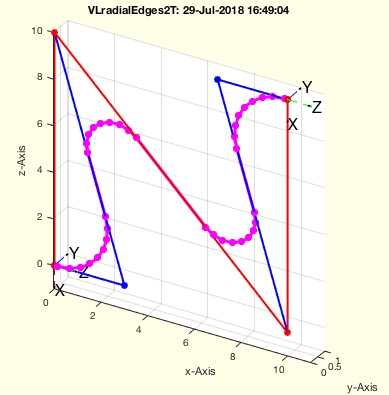% VLradialEdges2T(VL,R,TA,TB) - returns a vertex list (VL) with rounded/broken wrt to start and end frames% (by Tim Lueth, VLFL-Lib, 2018-JUL-29 as class: ANALYZING PROCEDURES)% % VLradialEdges uses VLtangentcirc uses PLtangentcirc% Replaces edges by a radial curve. The desired radius can be reduced % automatically if necessary. It is possible to maximize the radius % automatically.% In case that R is negative; VLbreakEdges is used instead (Status of: % 2018-07-29)% % Introduced first in SolidGeometry 4.2% % See also: PLradialEdges, SGcontourtube, RLofEulerInterpolation, % VLinsertEulerSteps, VLradialEdges, TofPez, PLtangentcirc, % VLtangentcirc, SGbreakCorners, SGradialCorners% % NVL=VLradialEdges2T(VL,[R,TA,TB])% === INPUT PARAMETERS ===% VL: Vertex list nx3% R: Radius; default is 1% TA: Rotation Matrix for Start Point% TB: Rotation Matrix for End Point% === OUTPUT RESULTS ======% NVL: New vertex list% % EXAMPLE: % VLradialEdges2T(VLsample(5),+3,TofR(rot(0,pi/2,0)),rot(0,pi/1.2,0))% VLradialEdges2T(VLsample(5),-3,TofR(rot(0,pi/2,0)),rot(0,pi/1.2,0))% % See also: PLradialEdges, SGcontourtube, RLofEulerInterpolation, % VLinsertEulerSteps, VLradialEdges, TofPez, PLtangentcirc, % VLtangentcirc, SGbreakCorners, SGradialCorners% % % Copyright 2018 Tim C. Lueth
###### PLtransform(PLA, PLB, sl,CL);- transform one PL into another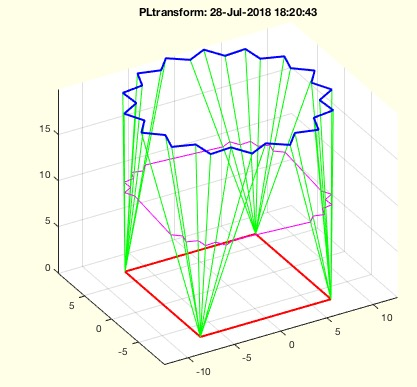% PLtransform(PLA, PLB, sl,CL); - transform one PL into another% (by Tim Lueth, VLFL-Lib, 2018-JUL-28 as class: CLOSED POLYGON LISTS)% % Introduced first in SolidGeometry 4.2% % See also: SGof2CPLz, FLofPLcorrelation, PLcorrelate, CPLcorrelate% % PLtransform(PLA,PLB,[sl,CL]);% === INPUT PARAMETERS ===% PLA: Point list A% PLB: Point list B% sl: percentage between 0==PLA and 1==PLB% CL: Correlation List, see PLcorrelate% % EXAMPLE: % PLtransform(CPLofPL(PLcircle(10,4)),CPLofPL(PLstar(10)),0.5)% PLtransform(CPLofPL(PLstar(10)),CPLofPL(PLcircle(10,4)),0.2)% % See also: SGof2CPLz, FLofPLcorrelation, PLcorrelate, CPLcorrelate% % % Copyright 2018 Tim C. Lueth
###### DeepL- just opens the browser with the DeepL translation tool% DeepL - just opens the browser with the DeepL translation tool % (by Tim Lueth, VLFL-Lib, 2018-JUL-28 as class: AUXILIARY PROCEDURES)% % Introduced first in SolidGeometry 4.2% % See also: SGgrabcad% % DeepL% % EXAMPLE: Just try: DeepL% % See also: SGgrabcad% % % Copyright 2018 Tim C. Lueth
###### SGTbaseunderSG(SGR)- creates a solid as base plated under a given solid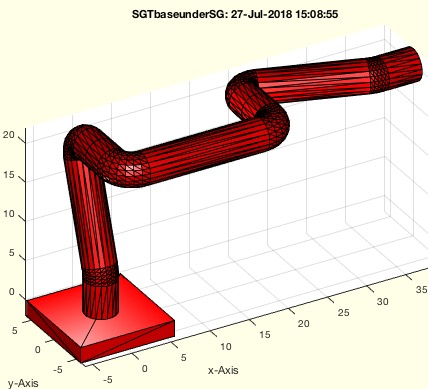% SGTbaseunderSG(SGR) - creates a solid as base plated under a given solid% (by Tim Lueth, VLFL-Lib, 2018-JUL-27 as class: KINEMATICS AND FRAMES)% % Introduced first in SolidGeometry 4.2% % See also: SGplateunder% % [SG,SGC]=SGTbaseunderSG(SGR)% === INPUT PARAMETERS ===% SGR: Solid or Cell list of SOlids% === OUTPUT RESULTS ======% SG: Solid under the first Element of SG% SGC: Cell list with SG as now first element% % EXAMPLE: % SGTofDHset([20 0 pi/2 0;0 20 pi 0; 0 20 0 pi/2]); A=ans% SGTbaseunderSG(A);% % See also: SGplateunder% % % Copyright 2018 Tim C. Lueth
###### permutevector(ps1,ps2,ps3)- creates vector list based on parametersets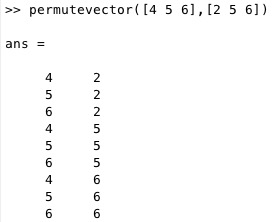% permutevector(ps1,ps2,ps3) - creates vector list based on parametersets% (by Tim Lueth, VLFL-Lib, 2018-JUL-26 as class: AUXILIARY PROCEDURES)% % Introduced first in SolidGeometry 4.2% % See also: publishTL, testfunctTL% % VL=permutevector([ps1,ps2,ps3])% === INPUT PARAMETERS ===% ps1: paraneter set 1% ps2: paraneter set 2% ps3: paraneter set 3% === OUTPUT RESULTS ======% VL: Value vector list% % EXAMPLE: % permutevector([4 5 6],[2 5 6],[9 8])% permutevector([4 5 6],[2 5 6])% permutevector([4 5 6])% % See also: publishTL, testfunctTL% % % Copyright 2018 Tim C. Lueth
###### forwkinrplan3(d1,a2,a3,WL)- forward kinematic of a planar rz 2 arm rotating around an arm z axis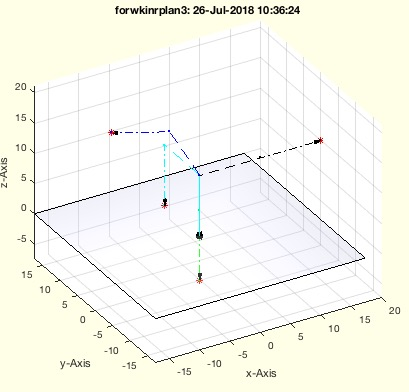% forwkinrplan3(d1,a2,a3,WL) - forward kinematic of a planar rz 2 arm rotating around an arm z axis% (by Tim Lueth, VLFL-Lib, 2018-JUL-26 as class: KINEMATICS AND FRAMES)% % Introduced first in SolidGeometry 4.2% % See also: invkinrplan3, SGTofDHset, SGTofDenavitHartenberg% % [VL3,VL2,VL1,RL3,TL3]=forwkinrplan3(d1,a2,a3,[WL])% === INPUT PARAMETERS ===% d1: distance of link 1% a2: width of link 2% a3: width of link 3% WL: Linst of angle (n x 3)% === OUTPUT RESULTS ======% VL3: Coordinates of link3% VL2: Coordinates of link2% VL1: Coordinates of link1% RL3: Rotation matrix at link 3 [3,3,n]% TL3: HT matrix at link 3 [4,4,n]% % EXAMPLE: % forwkinrplan3(10,10,10,[0 0 0; pi pi/3 pi/4;pi/3 -pi/3 pi/3; pi/2 0 % pi/2 ])% % See also: invkinrplan3, SGTofDHset, SGTofDenavitHartenberg% % % Copyright 2018 Tim C. Lueth
###### invkinrplan3(d1,a2,a3,VL)- returns the angles for a rotating planar two arm kinematic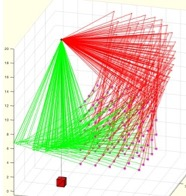% invkinrplan3(d1,a2,a3,VL) - returns the angles for a rotating planar two arm kinematic % (by Tim Lueth, VLFL-Lib, 2018-JUL-26 as class: KINEMATICS AND FRAMES)% % This fnctn contains the solution for a Jaco-Style 3DoF Kinematic % (Status of: 2018-07-27)% % Introduced first in SolidGeometry 4.2% % See also: forwkinrplan3, invkinplan2% % [aW,aU,bW,bU,ri,VL]=invkinrplan3(d1,a2,a3,[VL])% === INPUT PARAMETERS ===% d1: Height of the base% a2: length of link 2% a3: length of link 3% VL: Vertex list [n x 3]% === OUTPUT RESULTS ======% aW: Set of Configuration A (ellbow up)% aU: Set of Configuration B (ellbow down)% bW: Set of Configuration C (reverse ellbow up)% bU: Set of Configuration D (reverse (ellbow down)% ri: row index of VL for real solutions% VL: Vertex list if created by this fnctn% % EXAMPLE: % invkinrplan3(20,15,10,permutevector(2:2:10,2:2:10,0));% % See also: forwkinrplan3, invkinplan2% % % Copyright 2018 Tim C. Lueth
###### subfig (p)- creates subfigures on the screen similar to subplot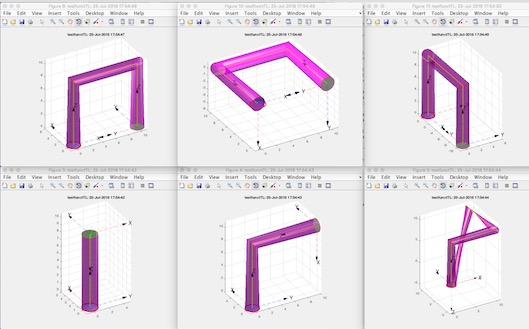% subfig (p) - creates subfigures on the screen similar to subplot% (by Tim Lueth, VLFL-Lib, 2018-JUL-25 as class: VISUALIZATION)% % Introduced first in SolidGeometry 4.2% % See also: copyfig% % subfig([p])% === INPUT PARAMETERS ===% p: position similar to subplot % % EXAMPLE: % for i=1:4; SGfigure(SGbox([30,20,10])); view(-30,30);end% for i=1:4; SGfigure(SGbox([30,20,10])); view(-30,30); copyfig; end% for i=1:4; SGfigure(SGbox([30,20,10])); view(-30,30); copyfig; % subfig(i); end% % See also: copyfig% % % Copyright 2018 Tim C. Lueth
###### testfunctTL(Eterm,)- testing macro that executes creates a list of different parametertests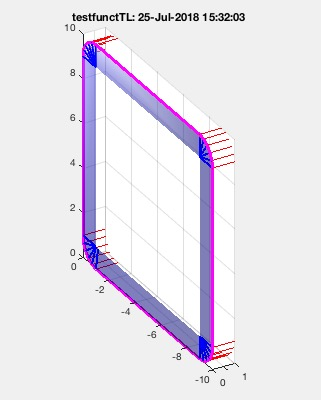% testfunctTL(Eterm,) - testing macro that executes creates a list of different parametertests% (by Tim Lueth, VLFL-Lib, 2018-JUL-25 as class: AUXILIARY PROCEDURES)% % uses evalin('caller',eterm) for execution to be able to use the defined % variables in the command workspace (Status of: 2018-07-28)% % Introduced first in SolidGeometry 4.2% % See also: publishTL, permutevector% % testfunctTL(Eterm,[])% === INPUT PARAMETERS ===% Eterm: fnctn string; Variables are listed as "#1" ,"#2, "%3"% % EXAMPLE: % testfunctTL('SGcontourtube2(PLcircle(1),VLsample(#1));',[2 3 8 12 13 14 % 20 21 22]);% testfunctTL('[#1 #2 #3]',[1 2 3],[4 5],[6 7])% testfunctTL('VLradialEdges(VLsample(#1))',2:3)% testfunctTL('VLedgeNormal(VLradialEdges(CVLofVL(VLsample(#1))));',[3 7 % 8 12 13 14 20 21 22]);% % See also: publishTL, permutevector% % % Copyright 2018 Tim C. Lueth
###### SGcontourtube2(CPL,VL)- extrudes a CPL along a path given as CVL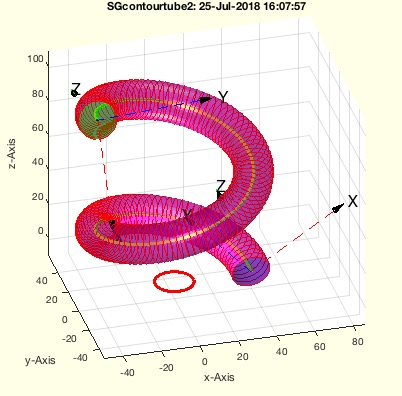% SGcontourtube2(CPL,VL) - extrudes a CPL along a path given as CVL% (by Tim Lueth, VLFL-Lib, 2018-JUL-24 as class: SURFACES)% % =======================================================================% OBSOLETE (2018-08-17) - USE 'SGofCPLtransT' INSTEAD% =======================================================================% % Improved Version of SGcontourtube% Powerful procedure to extrude PLs [nx2] anlog a path (Status of: % 2018-08-17)% % Introduced first in SolidGeometry 4.2% % See also: [ SGofCPLtransT ] ; TLofCVL, SGofCPLCVLR, SGcontourtube, % SGofCPLtransT, SGof2T, SGof2SGT, RLofEulerInterpolation, % VLinsertEulerSteps, VLradialEdges, TofPez% % [SG,FLS,FLE,FLW,TS,TE]=SGcontourtube2(CPL,VL)% === INPUT PARAMETERS ===% CPL: Closed point list [nx2]% VL: Vertex list [nx3]% === OUTPUT RESULTS ======% SG: Solid geometry% FLS: FL of starting surface% FLE: FL of ending surface% FLW: FL of the wall% TS: Starting Frame% TE: End Frame% % EXAMPLE: % VL=VLhelix(40,100,3*pi); % SGcontourtube(PLcircle(10),VL)% SGcontourtube2(PLcircle(10),VL)% % See also: [ SGofCPLtransT ] ; TLofCVL, SGofCPLCVLR, SGcontourtube, % SGofCPLtransT, SGof2T, SGof2SGT, RLofEulerInterpolation, % VLinsertEulerSteps, VLradialEdges, TofPez% % % Copyright 2018 Tim C. Lueth
###### Rofez(ezz,ystab)- returns a rotation matrix based on a list of ez vector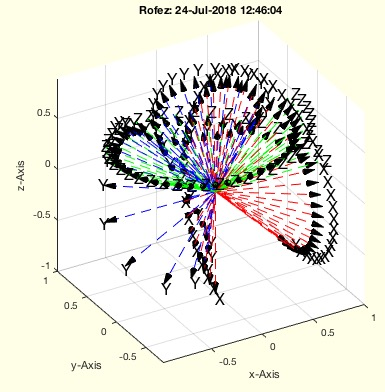% Rofez(ezz,ystab) - returns a rotation matrix based on a list of ez vector% (by Tim Lueth, VLFL-Lib, 2018-JUL-23 as class: ANALYTICAL GEOMETRY)% % could be improved in combination with VLedgeNormal (Status of: % 2018-07-24)% % Introduced first in SolidGeometry 4.2% % See also: VLedgeNormal% % R=Rofez(ezz,[ystab])% === INPUT PARAMETERS ===% ezz: ez vector or list of ez vectors% ystab: true== fix y-axis; false== fix x-axis; default is true;% === OUTPUT RESULTS ======% R: Rotation matrix or vector of rotation matrices% % EXAMPLE: % Rofez([0 0 1]% Rofez([0 0 1;0 0 -1])% % See also: VLedgeNormal% % % Copyright 2018 Tim C. Lueth
###### SGTofDHset(DHS,r,g)- returns the solids for a kinematic chain defined by DH parameter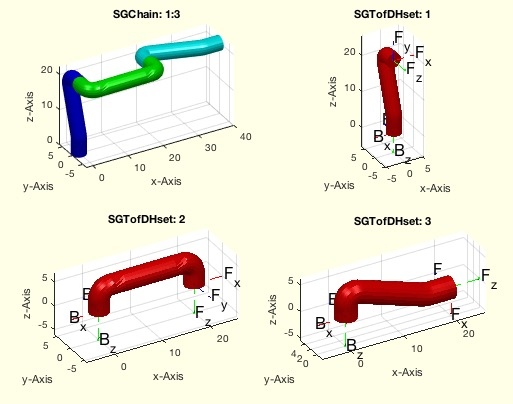% SGTofDHset(DHS,r,g) - returns the solids for a kinematic chain defined by DH parameter% (by Tim Lueth, VLFL-Lib, 2018-JUL-20 as class: KINEMATICS AND FRAMES)% % Introduced first in SolidGeometry 4.2% % See also: SGof2T, SGTset, SGTofDenavitHartenberg, SGTchain% % [SG,Ti,SGN,Tii,E]=SGTofDHset(DHS,[r,g])% === INPUT PARAMETERS ===% DHS: Denavit-Hartenberg Parameter [nx3] [d a wx] or [d a xw xy]% r: optional diameter for tube% g: optional offset for phiz% === OUTPUT RESULTS ======% SG: Cell list of Solid Geoemtries% Ti: Cell ist of Symbolic Equation% SGN: Compiled Solid using the kinematic chain% Tii: Cell list of complete symbolic equations% E: Final movement matrix of the TCP% % EXAMPLE: SGTofDHset([10 0 pi/2])% SGTofDHset([20 0 pi/2;0 20 pi;10 0 pi/2; 3 0 pi/4; 3 0 pi/4],1,pi/10); % SG=ans% SGTofDHset([20,0,pi/2 0;0 20 pi*0.99 0;0 20 0 pi/2]) % including % roation around y% SGTofDHset([20 0 pi/2 0; 0,20,pi 0;0 10 0 pi/2;10 0 pi/4 0; 10 0 -pi/4 % 0;10 0 0 0])% % See also: SGof2T, SGTset, SGTofDenavitHartenberg, SGTchain% % % Copyright 2018 Tim C. Lueth
###### invkinplan2(l1,l2,PL)- returns the inverse kinematic for a planr 2 arm robot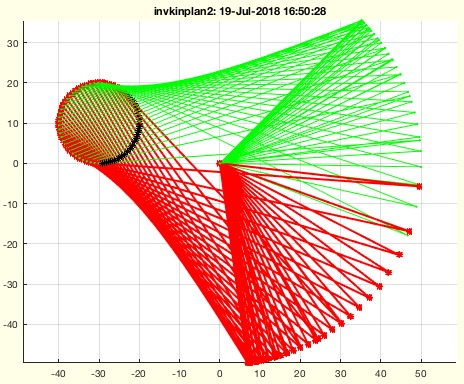% invkinplan2(l1,l2,PL) - returns the inverse kinematic for a planr 2 arm robot% (by Tim Lueth, VLFL-Lib, 2018-JUL-19 as class: MECHANICAL PROCEDURES)% % This is just an example how to implement the results of % exp_2018_07_19_invers_kin.mlx into a numeric fnctn. It containts the % closed analytical inverse kinematic for 2 swinging arms. This fnctn can % be used to simulate the movement of fourbar linkages (wrt. Franz % Irlinger)% If PL is empty, the workspace is analyzed by creating a squared map of % points% PL can also be a triple [r x y] of a circle with radius r at position x % and y. (Status of: 2018-07-19)% % Introduced first in SolidGeometry 4.2% % See also: exp_2018_07_19_invers_kin.mlx% % [W,U,B1,B2,rs,PL]=invkinplan2(l1,l2,[PL])% === INPUT PARAMETERS ===% l1: length first link% l2: length second link% PL: Point list (n x 2)% === OUTPUT RESULTS ======% W: Angle Pair [n x 2] for solution 1% U: Angle Pair [n x 2] for solution 2% B1: Endpoint of Arm 1 for solution 1% B2: Endpoint of Arm 1 for solution 2% rs: real solutions index; true ~ solution exist% PL: used Point list in case that PL was empty or a triple for a circle% % EXAMPLE: invkinplan2(25,20,[20 20; 25 25;27 27])% w=0:0.3:2*pi; w=w'; PL=30*[cos(w) sin(w)];% invkinplan2(20,11,PL)% w=0:0.3:2*pi; w=w'; PL=10*[cos(w) sin(w)]+[-30 +5];% invkinplan2(30,20,PL)% w=0:0.1:2*pi; w=w'; PL=10*[cos(w) sin(w)]+[-30 10]; % invkinplan2(50,80,PL);% invkinplan2(50,80); % Check work space% invkinplan2(50,80,[10 -40 10]); % Check a fourbar linkage% % See also: exp_2018_07_19_invers_kin.mlx% % % Copyright 2018 Tim C. Lueth
###### SGTofDenavitHartenberg(D,A,be,r,g)- returns from Denavit-Hartenberg values, a solid, frames and a symbolic description for DH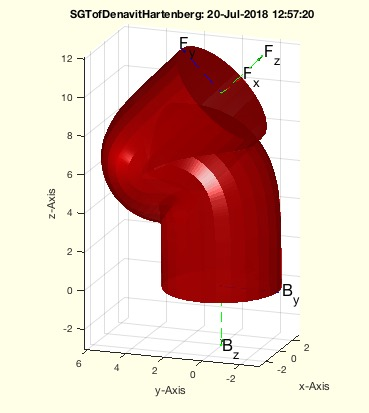% SGTofDenavitHartenberg(D,A,be,r,g) - returns from Denavit-Hartenberg values, a solid, frames and a symbolic description for DH% (by Tim Lueth, VLFL-Lib, 2018-JUL-13 as class: MODELING PROCEDURES)% % This fnctn returns for give parameter sets with respect to the % Denavit-Hartenberg (1955) notation:% d=displacement in z% a=displacement in x% b=rotation around x% a modern style link (shape along z trajectory)% 6Dof Roboter lassen sich aus praktischen Gründen (Nutzung und % Berechnung der inversen Kinematik sinnvoller Weise wie folgt entwerfen: % 1. Trennung von Hand und Roboter in je drei Achsen.% 2. Die 6. Achse ist eine möglichst kurze reine Z-Achse.% 3. Die 5. und 4. Achse erlaube eine möglichst kompakte Drehung auf % einer Kugeloberfläche% 4. Die 1. Achse ist eine Z-Achse die so hoch ist dass 2 und 3 optimale % arbeiten können% 5. Die 2 und 3 Achse ind quasi gleich lang. 3 ist kürzer weil 4 und 5 % und 6 noch dazu kommen.% Die inverse Kinematik wird so gelöst, dass ausgehend vom Zielpunkt % z-Achse 6 definiert ist % (Status of: 2018-07-20)% % Introduced first in SolidGeometry 4.2% % See also: SGTofDHset, SGof2T, SGTset, SGTchain% % [SGT,T]=SGTofDenavitHartenberg(D,A,be,[r,g])% === INPUT PARAMETERS ===% D: Distance in z% A: Distance in z% be: fixed rotation value around x-axis or [x-axis/yaxis]% r: optional Radius of the tube; default is max(D,A)/10% g: optional angle as offset for a rotation at the base; default is 0% === OUTPUT RESULTS ======% SGT: Solid Geoemtry including Frames% T: % % EXAMPLE: Different common robotics links:% SGTofDenavitHartenberg(10,0,0); % SGTofDenavitHartenberg(10,10,0);% SGTofDenavitHartenberg(10,0,pi/2);% SGTofDenavitHartenberg(0,10,pi);% SGTofDenavitHartenberg(10,0,pi/4,3);% SGTofDenavitHartenberg(10,0,pi/2,'',pi/2); % SGTofDenavitHartenberg (0,20,[0,pi/2]);% % See also: SGTofDHset, SGof2T, SGTset, SGTchain% % % Copyright 2018 Tim C. Lueth
###### gcahandleofSG- returns the handle to all patches of gca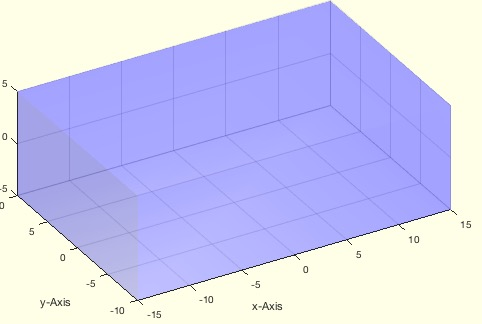% gcahandleofSG - returns the handle to all patches of gca% (by Tim Lueth, VLFL-Lib, 2018-JUL-10 as class: VISUALIZATION)% % This fnctn returns the handle to all patches in the current graphics % axis. I tcan be used to change color, transparency, or to create a SG. % (Status of: 2018-07-19)% % Introduced first in SolidGeometry 4.2% % See also: ispatch, SGofgca% % h=gcahandleofSG% === OUTPUT RESULTS ======% h: handle of all patches in gca% % EXAMPLE: % SGfigure; view(-30,30); SGplot(SGbox([30,20,10]));% setplotlight(gcahandleofSG,'b',0.2), show% % See also: ispatch, SGofgca% % % Copyright 2018 Tim C. Lueth
###### subsvar(f,sv,)- replaces several symbols in an equation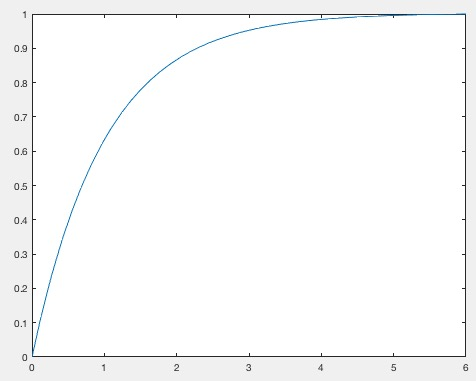% subsvar(f,sv,) - replaces several symbols in an equation% (by Tim Lueth, VLFL-Lib, 2018-JUN-29 as class: SYMBOLICS)% % This fnctn is required to substitute several symbols or terms in a % symbolic equation (Status of: 2018-06-29)% % Introduced first in SolidGeometry 4.2% % g=subsvar(f,sv,[])% === INPUT PARAMETERS ===% f: original term / equation% sv: array of symbols to substitute or [s1 v1; s2 z2];% === OUTPUT RESULTS ======% g: resulting term / equation% % EXAMPLE: % syms x(t) D C F m; dx=diff(x); ddx=diff(x,2);% f=dsolve(m*ddx+D*dx==0, x(0)==0,x(6)==1); sv=symvar(f)% subsvar(f,[D m],[1 1])% subsvar(f,[D 1;m 1])% % % Copyright 2018 Tim C. Lueth
###### VLFLvertexNormal(VL,FL)- returns the vertex normal for the vertices of a solid% VLFLvertexNormal(VL,FL) - returns the vertex normal for the vertices of a solid% (by Yilun Sun, VLFL-Lib, 2018-JUN-21 as class: ANALYTICAL GEOMETRY)% % This fnctn calculates the vertex normal from the average normal of the % surrounding facets weighted by the corresponding angles.% (Status of: 2018-06-22)% % Introduced first in SolidGeometry 4.2% % See also: PLnorm, PLELnorm, PLFLfaceNormal, VLnorm, VLFLnormf, % VLFLfaceNormal, VLedgeNormal, VLFLfaceAngles% % LITERATURE:% - Grit Thuermer and Charles A. Wuethrich (1998): "Computing vertex % normals from polygonal facets", Journal of Graphics Tools, Volume 3 % Issue 1, Mar 1998, Pages 43-46% % NVL=VLFLvertexNormal(VL,FL)% === INPUT PARAMETERS ===% VL: Vertex list% FL: Facet list% === OUTPUT RESULTS ======% NVL: Normal Vector List [nf x 3]% % EXAMPLE: % [VL,FL]=SGsample(4); VLFLvertexNormal(VL,FL);% [VL,FL]=SGsample(5); VLFLvertexNormal(VL,FL);% [VL,FL]=VLFLsample(11); VLFLvertexNormal(VL,FL)% % See also: PLnorm, PLELnorm, PLFLfaceNormal, VLnorm, VLFLnormf, % VLFLfaceNormal, VLedgeNormal, VLFLfaceAngles%
###### usedinEL(NEL)- returns a list of unique numbers and the number of use in the list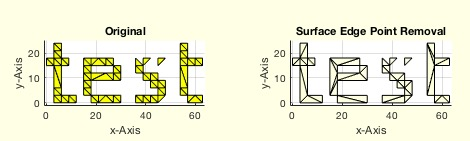% usedinEL(NEL) - returns a list of unique numbers and the number of use in the list% (by Tim Lueth, VLFL-Lib, 2018-JUN-02 as class: AUXILIARY PROCEDURES)% % Auxiliary fnctn for searching in non manifold or corrupted edge lists % (Status of: 2018-06-03)% % Introduced first in SolidGeometry 4.2% % See also: graphofEL, ELplot% % PIL=usedinEL(NEL)% === INPUT PARAMETERS ===% NEL: Any kind of list of integers, such as EL, FL% === OUTPUT RESULTS ======% PIL: Entry index list [Entry number]% % EXAMPLE: % CPLoftext('test'); CPL=ans; % Create contours% CPL=roundn(CPL,3); % Grid will create non manifold edges% SGfigure; CPLplot(CPL); [PL,EL]=PLELofCPL(CPL); % [PL,EL]=VLELshort(PL,EL); % removed doubled used points% EL=unique(EL,'rows','stable'); % remove doubled edges% EL=EL(EL(:,1)~=EL(:,2),:) % remove point type edges% usedinEL(EL)% TR=delaunayTriangulation(PL,EL);% SGfigure; VLFLplot(TR.Points,TR.ConnectivityList(isInterior(TR),:))% B.VL=VLaddz(TR.Points); B.FL=TR.ConnectivityList(isInterior(TR),:)% B=SGshort(B); % remove unused points% SGremsurfedgepoints(B)% % See also: graphofEL, ELplot% % % Copyright 2018 Tim C. Lueth
###### FEsort(FEL)- groups and sorts unsorted edge lists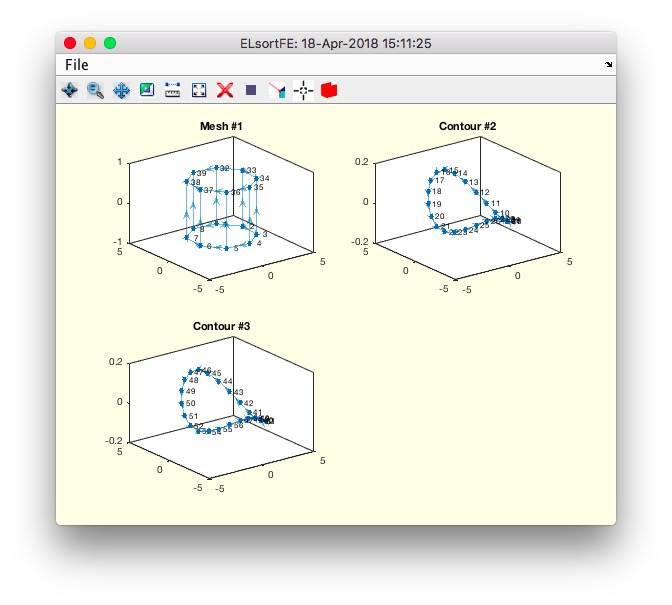% FEsort(FEL) - groups and sorts unsorted edge lists% (by Tim Lueth, VLFL-Lib, 2018-APR-18 as class: EDGE LISTS)% % =======================================================================% OBSOLETE (2018-04-18) - USE 'ELsortFE' INSTEAD% =======================================================================% % Same as ELsortFE% Feature Edge lists are unsorted and not directed. This fnctns separates % the edge lists into groups of meshes and contours and sorts contours % into one direction. There is no guarantee that it is the right % direction since the vertices are unknown to this fnctn. (Status of: % 2018-04-18)% % Introduced first in SolidGeometry 4.2% % [EL,CEL,mi]=FEsort(FEL)% === INPUT PARAMETERS ===% FEL: Unsorted edge list for instance a feature edge list% === OUTPUT RESULTS ======% EL: Edge list% CEL: Contour index list% mi: mesh index; false = contour, true is mesh% % EXAMPLE: % FEsort(FEofSG(SGsample(32)))% % % Copyright 2018 Tim C. Lueth
###### ELsortFE(FEL)- groups and sorts unsorted edge lists% ELsortFE(FEL) - groups and sorts unsorted edge lists% (by Tim Lueth, VLFL-Lib, 2018-APR-18 as class: EDGE LISTS)% % Feature Edge lists are unsorted and not directed. This fnctns separates % the edge lists into groups of meshes and contours and sorts contours % into one direction. There is no guarantee that it is the right % direction since the vertices are unknown to this fnctn. (Status of: % 2018-04-18)% % Introduced first in SolidGeometry 4.2% % See also: ELconnectedgroups, CELofEL% % [EL,CEL,mi]=ELsortFE(FEL)% === INPUT PARAMETERS ===% FEL: Unsorted edge list for instance a feature edge list% === OUTPUT RESULTS ======% EL: Edge list% CEL: Contour index list% mi: mesh index; false = contour, true is mesh% % EXAMPLE: % ELsortFE(FEofSG(SGsample(32)))% % See also: ELconnectedgroups, CELofEL% % % Copyright 2018 Tim C. Lueth
###### PLchamfer(z,ph,sh,d)- returns a point list for chamfer shape functions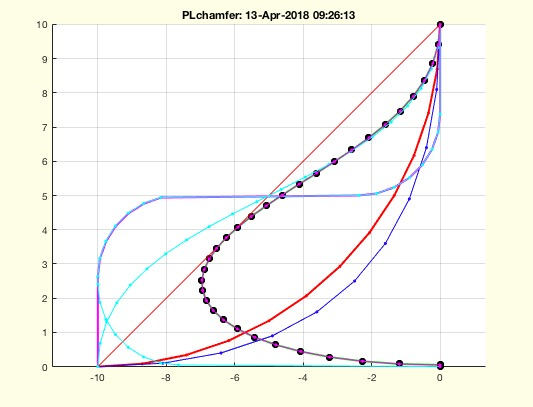% PLchamfer(z,ph,sh,d) - returns a point list for chamfer shape fnctns% (by Tim Lueth, VLFL-Lib, 2018-APR-11 as class: MODELING PROCEDURES)% % written in Al-Dakhla, Marocco, % From a z value and a phase distance, several different curves are % calculate that can be used to create chamfers of 2.5D solids using % fnctn SGchamfer% line - direct chamfer% circ - 90 degree chamfer% circ2 - 90/-90 degree chamfer% circ3 - 90/-90/90 degree chamfer% bezier - 90 degree Bezier spline% bezier2 - 90/-90 degree Bezier spline% bezier3 - 90/-90/90 degree Bezier spline% mattheck - zugdreieck-methode nach mattheck ~ tan(0..1.32)% (Status of: 2018-04-13)% % Introduced first in SolidGeometry 4.2% % See also: SGchamfer% % PL=PLchamfer([z,ph,sh,d])% === INPUT PARAMETERS ===% z: height% ph: chamfer thickness% sh: shape such as 'line', 'circ'% d: value for straight end distance% === OUTPUT RESULTS ======% PL: Point list for a chamfer design% % EXAMPLE: % PLchamfer(10,-10)% PLchamfer(10,-10,'circ')% PLchamfer(10,-10,'bezier')% % See also: SGchamfer% % % Copyright 2018 Tim C. Lueth
###### SGfiguremovie(fname,mtime,angle,ofs)- creates a movie from a simple rotating view point in MP4 Format% SGfiguremovie(fname,mtime,angle,ofs) - creates a movie from a simple rotating view point in MP4 Format% (by Tim Lueth, VLFL-Lib, 2018-MÄR-12 as class: VISUALIZATION)% % This fnctn is also helpful to learn to create movies from figure % snapshots% 2018-04-17 Default format changed from 'AVI' to 'MPEG-4'% (Status of: 2018-04-17)% % Introduced first in SolidGeometry 4.2% % See also: imageVideoWrite, SGfigurevideo, SGfigure, SGtitle, % SGfigureannotation, imageVideoEndtitle, imageVideoFrames, % imageVideoTextPage, imageVideoTitle, videoCopyCutMovies, videoCopyFrames% % SGfiguremovie([fname,mtime,angle,ofs])% === INPUT PARAMETERS ===% fname: file name on desktop; default is 'SGfiguremovie.avi'% mtime: movie time; default is 6 seconds% angle: turning angle; default is [10 370]% ofs: offset to start angle; default is 0% % EXAMPLE: % SGsample(25);% SGfiguremovie% SGfiguremovie('',3)% SGfiguremovie('',3,[-20 20])% % % See also: imageVideoWrite, SGfigurevideo, SGfigure, SGtitle, % SGfigureannotation, imageVideoEndtitle, imageVideoFrames, % imageVideoTextPage, imageVideoTitle, videoCopyCutMovies, videoCopyFrames% % % Copyright 2018 Tim C. Lueth
###### SGfigureannotation(str,bc,sz,sl,hl)- creates a simple help text annotation into the current figure% SGfigureannotation(str,bc,sz,sl,hl) - creates a simple help text annotation into the current figure% (by Tim Lueth, VLFL-Lib, 2018-MÄR-12 as class: USER INTERFACE)% % Used in fnctns such as SGTui (Status of: 2018-03-12)% % Introduced first in SolidGeometry 4.2% % See also: SGfigure, SGtitle% % h=SGfigureannotation(str,[bc,sz,sl,hl])% === INPUT PARAMETERS ===% str: string% bc: backgoundcolor; default is unchanged% sz: text size; default is 10% sl: starting position from bottom; default is 0.97%% hl: height of annotation; default is 1-sl% === OUTPUT RESULTS ======% h: handle to annotation% % EXAMPLE: % SGfigure;% SGfigureannotation('This is a simple help text','y',16,0.9)% % See also: SGfigure, SGtitle% % % Copyright 2018 Tim C. Lueth
###### SGchecklist (A,ni)- performs a visible check using a checklist for a given solid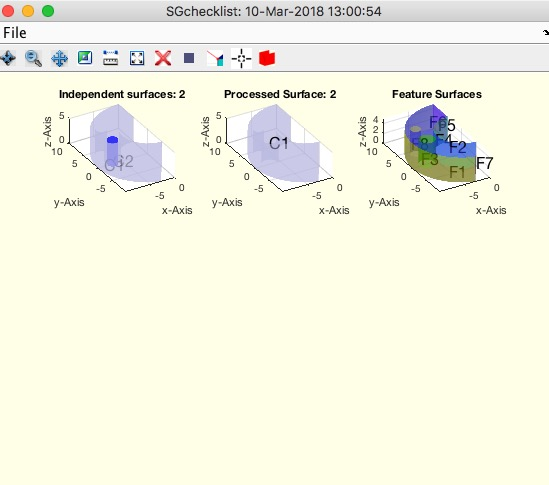% SGchecklist (A,ni) - performs a visible check using a checklist for a given solid % (by Tim Lueth, VLFL-Lib, 2018-MÄR-10 as class: CHECKING PROCEDURES)% % Checking a surface is a challenging task. If a complex surface is % sound, there is nothing to do. In case that a solid, produces created % by a voxel mode or a CSG model is corrupted, many problems occur. An % there is almost no chance to see the problem in an isolated manner % (Status of: 2018-03-10)% % Introduced first in SolidGeometry 4.2% % See also: SGsurfaces, FSofSG, CVLfreeBoundaryVLFL, VLFLcloseopenfacets% % % SGchecklist(A,[ni])% === INPUT PARAMETERS ===% A: Solid% ni: % % EXAMPLE: % SGchecklist(SGsample(25))% % See also: SGsurfaces, FSofSG, CVLfreeBoundaryVLFL, VLFLcloseopenfacets% % % % Copyright 2018 Tim C. Lueth
###### SGplot4(SG,)- plots a solid in 4 views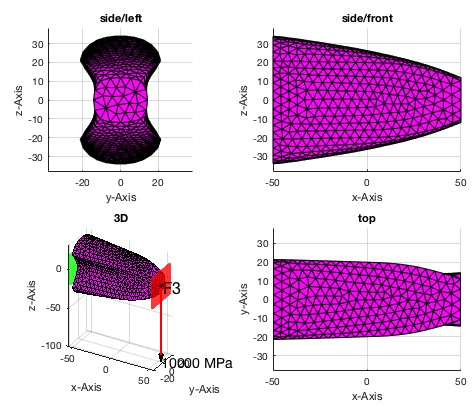% SGplot4(SG,) - plots a solid in 4 views% (by Tim Lueth, VLFL-Lib, 2018-MÄR-08 as class: VISUALIZATION)% % Introduced first in SolidGeometry 4.2% % See also: VLFLplot4, SGplot% % SGplot4(SG,[])% === INPUT PARAMETERS ===% SG: Solid Geometry% % EXAMPLE: SGplot4(SGsample(25),'m')% % See also: VLFLplot4, SGplot% % % Copyright 2018 Tim C. Lueth
###### VLFLremsurfedgepoints(VL,FL,alpha,minf)- returns a surface model without edge points and surface points that are inside of a surface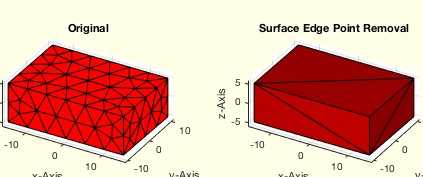% VLFLremsurfedgepoints(VL,FL,alpha,minf) - returns a surface model without edge points and surface points that are inside of a surface% (by Tim Lueth, VLFL-Lib, 2018-MÄR-06 as class: SURFACES)% % Calls fnctn as SGremsurfedgepoints. This fnctn is useful if a % tetrahedron model (pde mesh) is converted into a surface model, or a % solid is bent somehow. Afterwards there a surfaces that consist of many % vertices that are in the same plane. This fnctn analyses the surface % and retesselate all surface based only on the boundary vertices. % (Status of: 2018-03-06)% % Introduced first in SolidGeometry 4.2% % See also: VLFLremsurfpoints, SGremsurfpoints, SGofpdemodel, % SGremsurfedgepoints% % [VLN,FLN,FLNorg,FSi]=VLFLremsurfedgepoints(VL,FL,[alpha,minf])% === INPUT PARAMETERS ===% VL: Vertex list of surface model% FL: Facet list of surface model% alpha: alpha angle for feature surface; default is 0.01% minf: minimum facets per face; default is 4% === OUTPUT RESULTS ======% VLN: New vertex list surface model with reduced vertices% FLN: New facet list surface model with reduced vertices% FLNorg: Reduced Facet list based on the original vertex list% FSi: Feature surface index list% % EXAMPLE: Remove surface and edge points% A=SGbox([30,20,10])% model=pdemodelofSG(A,5)% SGofpdemodel(model); B=ans; VL=B.VL; FL=B.FL;% VLFLremsurfedgepoints(B.VL,B.FL)% % See also: VLFLremsurfpoints, SGremsurfpoints, SGofpdemodel, % SGremsurfedgepoints% % % Copyright 2018 Tim C. Lueth
###### ELremove(EL,indl)- removes an index from an unsorted edge list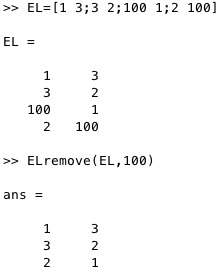% ELremove(EL,indl) - removes an index from an unsorted edge list% (by Tim Lueth, VLFL-Lib, 2018-MÄR-06 as class: EDGE LISTS)% % throws an error if an index list element is not part of the edge list % (Status of: 2018-03-06)% % Introduced first in SolidGeometry 4.2% % See also: ELsort% % ELN=ELremove(EL,indl)% === INPUT PARAMETERS ===% EL: original unsorted edge list% indl: index to be removed, or index list% === OUTPUT RESULTS ======% ELN: new unsorted edge list without the index% % EXAMPLE: remove one index% EL=[1 3;3 2;100 1;2 100]% ELremove(EL,100)% ELremove(EL,[1 2])% % See also: ELsort% % % Copyright 2018 Tim C. Lueth
###### SGremsurfedgepoints(SG,alpha,minf)- returns a surface model without edge points and surface points that are inside of a surface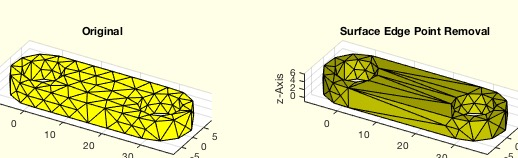% SGremsurfedgepoints(SG,alpha,minf) - returns a surface model without edge points and surface points that are inside of a surface% (by Tim Lueth, VLFL-Lib, 2018-MÄR-06 as class: SURFACES)% % This fnctn is useful if a tetrahedron model (pde mesh) is converted % into a surface model, or a solid is bent somehow. Afterwards there a % surfaces that consist of many vertices that are in the same plane. This % fnctn analyses the surface and retesselate all surface based only on % the boundary vertices. (Status of: 2018-03-06)% % Introduced first in SolidGeometry 4.2% % See also: VLFLremsurfpoints, SGremsurfpoints, SGofpdemodel% % [SGN,FLN,FSi]=SGremsurfedgepoints(SG,[alpha,minf])% === INPUT PARAMETERS ===% SG: Solid Geometry surface model% alpha: alpha angle for feature surface; default is 0.01% minf: minimum facets per face; default is 4% === OUTPUT RESULTS ======% SGN: New surface model with reduced vertices and faces% FLN: Reduced Facet list based on the original vertex list% FSi: Feature surface index list% % EXAMPLE: Remove surface points% A=SGlinkage(5,30,5)% model=pdemodelofSG(A,.5)% SGofpdemodel(model); B=ans% SGremsurfedgepoints(B)% % See also: VLFLremsurfpoints, SGremsurfpoints, SGofpdemodel% % % Copyright 2018 Tim C. Lueth% SGplotsurfaceload (SG,fixfacet,loadfacet,loadvec) - plots the surface load of a solid geometry% (by Tim Lueth, VLFL-Lib, 2018-MÄR-03 as class: FEM/PDE)% % This fnctn displays the surfaceload sitation that is used as input for % the fnctns:% pdesolvesurfaceload% pdestressstatic% SGshapeOptiCAO% % (Status of: 2018-03-23)% % Introduced first in SolidGeometry 4.2% % See also: pdestressstatic, pdesolvesurfaceload, SGshapeOptiCAO% % SGplotsurfaceload(SG,[fixfacet,loadfacet,loadvec])% === INPUT PARAMETERS ===% SG: Solid Geometry% fixfacet: list of fix Feature surfaces (green)% loadfacet: list of loaded Feature surfaces (red)% loadvec: vector of load per Feature surface% === PROPERTY NAMES =====% 'FixedFaceIndices' : the face indices of the faces which are fixed% 'LoadFaceIndices' : the face indices of the faces which are loaded% 'Load' : the load list according to LoadFaceIndices(unit of load is N)% % EXAMPLE: % A=SGbox([100,40,40]); SGfigure; % subplot(1,2,1); FSplot(A); view(30,30); % subplot(1,2,2); SGplotsurfaceload(A,[4 5],3,1e4); view(30,30);% % SGfigure; h=SGplot(A); setplotlight(h,'w',0.8); % SGplotsurfaceload(A,4,3,[0 -1e4 0]); view(30,30)% % SGfigure; h=SGplot(A); setplotlight(h,'w',0.8); view(30,30); % SGplotsurfaceload (A,'FixedFaceIndices',4,...% 'LoadFaceIndices',[3 3 NaN 5],'Load',[0 0 -4; 0 0 6; NaN NaN NaN;0 -2 % 0])% % % % See also: pdestressstatic, pdesolvesurfaceload, SGshapeOptiCAO% % % Copyright 2018 Tim C. Lueth
###### VLFLremsurfpoints(VL,FL,)- returns a surface model without surface points that are inside of a surface - boundary/edge points are unchanged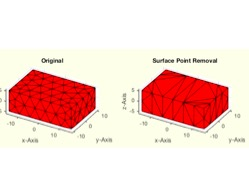% VLFLremsurfpoints(VL,FL,) - returns a surface model without surface points that are inside of a surface - boundary/edge points are unchanged% (by Tim Lueth, VLFL-Lib, 2018-MÄR-02 as class: SURFACES)% % Calls fnctn as SGremsurfpoints. This fnctn is useful if a tetrahedron % model (pde mesh) is converted into a surface model, or a solid is bent % somehow. Afterwards there a surfaces that consist of many vertices that % are in the same plane. This fnctn analyses the surface and retesselate % all surface based only on the boundary vertices. (Status of: 2018-03-02)% % Introduced first in SolidGeometry 4.2% % See also: VLFLremsurfpoints, SGremsurfpoints, SGofpdemodel% % [VLN,FLN,FLNorg,FSi]=VLFLremsurfpoints(VL,FL,[])% === INPUT PARAMETERS ===% VL: Vertex list of surface model% FL: Facet list of surface model% === OUTPUT RESULTS ======% VLN: New vertex list surface model with reduced vertices% FLN: New facet list surface model with reduced faces% FLNorg: Reduced Facet list based on the original vertex list% FSi: Feature surface index list% % EXAMPLE: Remove surface points% A=SGbox([30,20,10])% model=pdemodelofSG(A,5)% SGofpdemodel(model); B=ans; VL=B.VL; FL=B.FL;% SGremsurfpoints(B)% % See also: VLFLremsurfpoints, SGremsurfpoints, SGofpdemodel% % % Copyright 2018 Tim C. Lueth
###### SGremsurfpoints(SG,alpha)- returns a surface model without surface points that are inside of a surface - boundary/edge points are unchanged% SGremsurfpoints(SG,alpha) - returns a surface model without surface points that are inside of a surface - boundary/edge points are unchanged% (by Tim Lueth, VLFL-Lib, 2018-MÄR-02 as class: SURFACES)% % This fnctn is useful if a tetrahedron model (pde mesh) is converted % into a surface model, or a solid is bent somehow. Afterwards there a % surfaces that consist of many vertices that are in the same plane. This % fnctn analyses the surface and retesselate all surface based only on % the boundary vertices. (Status of: 2018-03-02)% % Introduced first in SolidGeometry 4.2% % See also: VLFLremsurfpoints, SGofpdemodel% % [SGN,FLN,FSi]=SGremsurfpoints(SG,[alpha])% === INPUT PARAMETERS ===% SG: Solid Geometry surface model% alpha: alpha angle for feature surface; default is 0.01% === OUTPUT RESULTS ======% SGN: New surface model with reduced vertices and faces% FLN: Reduced Facet list based on the original vertex list% FSi: Feature surface index list% % EXAMPLE: Remove surface points% A=SGbox([30,20,10])% model=pdemodelofSG(A,5)% SGofpdemodel(model); B=ans% SGremsurfpoints(B)% % See also: VLFLremsurfpoints, SGofpdemodel% % % Copyright 2018 Tim C. Lueth
###### SGofpdemodel(model)- returns a solid geometry surface model of a pde model% SGofpdemodel(model) - returns a solid geometry surface model of a pde model% (by Tim Lueth, VLFL-Lib, 2018-FEB-28 as class: FEM/PDE)% % the tetrahedron models of the model.Mesh are used to create a % tetrahedron triangulation. The freeboundary surface of this model is % used to create the surface model.% In contrast to tetramesh, the tetrahedrons in 3D pde models have 11 % vertices. (Status of: 2018-02-28)% % Introduced first in SolidGeometry 4.2% % See also: pdemodelofSG, SGtetramesh, SGremsurfpoints% % SG=SGofpdemodel(model)% === INPUT PARAMETERS ===% model: pde model created by pdemodelofSG% === OUTPUT RESULTS ======% SG: Solud Geometry surface model% % EXAMPLE: % A=SGlinkage(5,40,5)% model=pdemodelofSG(A,1)% SGofpdemodel(model)% % See also: pdemodelofSG, SGtetramesh, SGremsurfpoints% % % Copyright 2018 Tim C. Lueth
###### SGofFS(SG,alp,fsi,t,d,s)- returns a list of solid geometries that are attached to features surfaces% SGofFS(SG,alp,fsi,t,d,s) - returns a list of solid geometries that are attached to features surfaces % (by Tim Lueth, VLFL-Lib, 2018-FEB-28 as class: SURFACES)% % This fnctn is more ore less the same as SGofSurface, but the surfaces % are addressed as Feature Surfaces. This fnctn is an auxiliary fnctn to % create pretty (more visible) representations of surfaces (Status of: % 2018-02-28)% % Introduced first in SolidGeometry 4.2% % See also: SGofSurface, FSofSG% % [SGc,FSc]=SGofFS(SG,[alp,fsi,t,d,s])% === INPUT PARAMETERS ===% SG: Solid geometry% alp: alpha angle to calculate the feature surfaces; default is 1% fsi: feature surface list; default is all% t: thickness of the solid; default is 0.5 used in SGofSurface% d: distance from the surface; default is 0.3 used in SGofSurface% s: distance from the surface; default is 0.3 used in SGofSurface% % === OUTPUT RESULTS ======% SGc: Cell list of Solids related to fsi% FSc: Cell list of Vertex list and facet list of the FS related to fsi% % EXAMPLE: % A=SGlinkage(5,40,5)% SGofFS(A,[],[4 5],2);% % % See also: SGofSurface, FSofSG% % % Copyright 2018 Tim C. Lueth
###### SGshapeOptiCAO(SG,)- returns the optimized shape of a given structure based on biological growth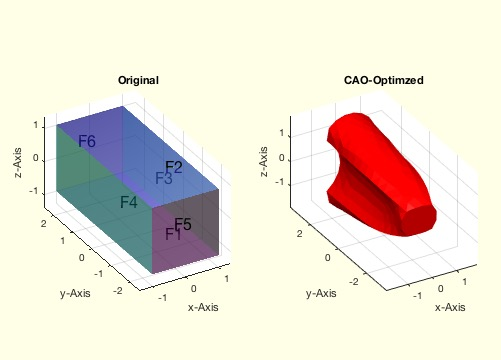% SGshapeOptiCAO(SG,) - returns the optimized shape of a given structure based on biological growth% (by Yilun Sun, VLFL-Lib, 2018-FEB-22 as class: FEM/PDE)% % The principle of the procedure is to allow the design proposal for the % desired component to grow into a shape of constant von Mises stress at % its surface.% It is possible to consider different load situations. In this case% (Status of: 2018-03-05)% % Introduced first in SolidGeometry 4.2% % See also: pdestressstatic, SGplotsurfaceload, FSplot% % LITERATURE:% - Harzheim, Lothar (2014): Strukturoptimierung - Grundlagen und % Anwendungen, Europa Lehrmittel.% % [SG,result,model,IterationAnalysis]=SGshapeOptiCAO(SG,[])% === INPUT PARAMETERS ===% SG: Solid Geometry of the initial design proposal(unit is mm)% === PROPERTY NAMES =====% 'MaxElementSize' : the maximal length of meshing element; def=1% 'LoadFaceIndices' : the face indices of the faces which are loaded% 'Load' : the load list according to LoadFaceIndices(unit of load is N)% 'FixedFaceIndices' : the face indices of the faces which are fixed% 'NonOptFaceIndices' : the face indices of the faces which are not to be % optimized% 'IterationNumber' : the maximal iterations for the optimization, def 30% === OUTPUT RESULTS ======% SG: Solid Geometry of the optimized structure% result: FEM anaysis result of the optimized structure(from PDE Toolbox)% model: pdemodel of the optimized structure(from PDE Toolbox)% IterationAnalysis: logged information of every optimization iteration% % EXAMPLE: % SG1 = SGbox([2,5,2]);% SGshapeOptiCAO(SG1,'MaxElementSize',0.4,'LoadFaceIndices',,...% 'Load',[0 0 -4],'FixedFaceIndices',6);% % [SG2,result,model,IA] = SGshapeOptiCAO(SG1,'MaxElementSize',0.4,...% 'LoadFaceIndices',,'Load',[0 0 -4],'FixedFaceIndices',5);% SGfigure(SG2); % % SGshapeOptiCAO(SG1,'MaxElementSize',0.4,...% 'LoadFaceIndices',[6 NaN 6],...% 'Load',[0 0 -4;NaN NaN NaN;0 0 5],'FixedFaceIndices',5);% % % See also: pdestressstatic, SGplotsurfaceload, FSplot% %
###### pdestressstatic(model,result,inputParser)- returns the calculated static stress inside a SG based on a pde model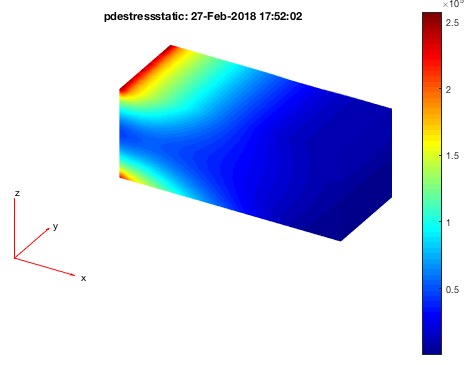% pdestressstatic(model,result,inputParser) - returns the calculated static stress inside a SG based on a pde model% (by Yilun Sun, VLFL-Lib, 2018-FEB-22 as class: FEM/PDE)% % This fnctn calculates the static von-Mises stress inside the SG. % By default, the model mesh is used for stress caluclation.% If only a few points are of interest, use a vertex list as optional % input parameter. In this case, the calculation is done for any points % (also outside of the grid) but there will by no graphical output. % (Status of: 2018-02-28)% % Introduced first in SolidGeometry 4.2% % See also: pdemodelofSG, pdeplotfaces, pdesolvesurfaceload, pdeplotresult% % [stresstensor,sVonMises]=pdestressstatic(model,result,[inputParser])% === INPUT PARAMETERS ===% model: pde-model (attention: modified / call by reference)% result: simulation result of fnctn pdesolvesurfaceload% inputParser: see Property Names% === PROPERTY NAMES =====% 'FaceAlpha' : Alpha value, default is 1% 'Points' : Vertex list [nx3], default is empty% 'Plot' : 'on' or 'off', default is 'off'% === OUTPUT RESULTS ======% stresstensor: List of stress tensors% sVonMises: static von-Mises stress% % EXAMPLE: Simple Load Example:% A=SGbox([100,40,40])% model=pdemodelofSG(A,5);% [result, model]=pdesolvesurfaceload(model,4,2,1e4)% pdeplotresult(model,result)% pdestressstatic(model,result)% % See also: pdemodelofSG, pdeplotfaces, pdesolvesurfaceload, pdeplotresult%
###### SGofCPLrota(CPL,alp,mov,amin)- returns a solid geometry by rotateing a closed x z contour around z axis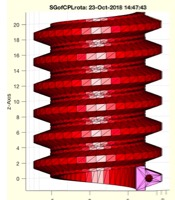% SGofCPLrota(CPL,alp,mov,amin) - returns a solid geometry by rotateing a closed x z contour around z axis% (by Tim Lueth, VLFL-Lib, 2017-OKT-18 as class: SURFACES)% % Introduced first in SolidGeometry 4.2% % [VL,FL]=SGofCPLrota(CPL,[alp,mov,amin])% === INPUT PARAMETERS ===% CPL: Closed contour in [x z]% alp: sweeping angle% mov: false = cut; negative x values; true=move to positive% amin: angle offset; default is 0% === OUTPUT RESULTS ======% VL: Vertex list or SG% FL: Facet list% % EXAMPLE: SGofCPLrota(CPLsample(31),1.5*pi);% SGofCPLrota(PLcircle(10),1.5*pi,false);% SGofCPLrota(PLcircle(10),1.5*pi,true);% % % Copyright 2017-2018 Tim C. Lueth